# Intervention Image 其他圖片處理

## cache() 快取

``````// 試著讀取快取的圖片
\$img = Image::cache(function(\$image) {
// 讀取本機圖片，將圖片 resize 至 300x200，並作灰階處理
return \$image->make('public/foo.jpg')
->resize(300, 200)
->greyscale();
});
``````

## canvas() 建立圖片畫布

``````// 建立畫布
\$img = Image::canvas(300, 100);
``````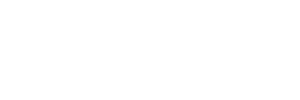Image::canvas(300, 100);

``````// 建立畫布，並使用 #ff0000 顏色當作背景
\$img = Image::canvas(32, 32, '#ff0000');
``````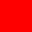Image::canvas(32, 32, ‘#ff0000’);

## ellipse() 畫橢圓

``````// 建立顏色為 #ddd，寬度 300px，高度 100px 的畫布
\$img = Image::canvas(300, 100, '#ddd');

// 在畫布中畫出寬度 25px，高度 60px，位置在 (50,50) ，顏色為 #0000ff 的橢圓
\$img->ellipse(25, 60, 50, 50, function (\$draw) {
\$draw->background('#0000ff');
});
``````\$img->ellipse(25, 60, 50, 50) color #0000ff

## exif() 取得圖片 exif 資訊

``````// 取得所有 exif 資訊
\$data = Image::make('public/foo.jpg')->exif();

// 讀取鏡頭 Model
\$name = Image::make('public/foo.jpg')->exif('Model');
``````

## filesize() 檔案大小

``````// 建立圖片實例
\$img = Image::make('public/foo.jpg');

// 取得檔案大小
\$size = \$img->filesize();
``````

## fill() 填滿顏色或圖片

``````// 建立畫布，寬度 800px 高度 600px
\$img = Image::canvas(800, 600);

// 填滿 #ff5500 顏色
\$img->fill('#ff5500');
``````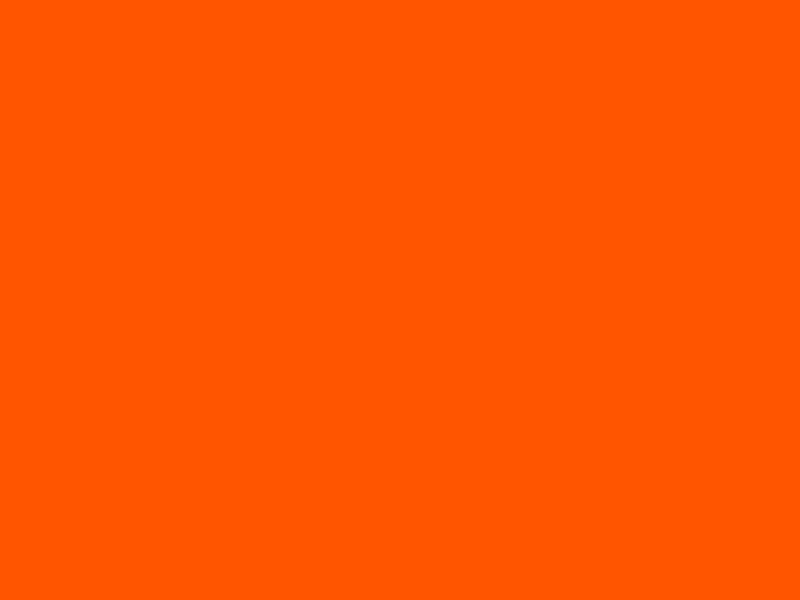\$img->fill('#ff5500’);

``````// 填滿圖片
\$img->fill('tile.png');
``````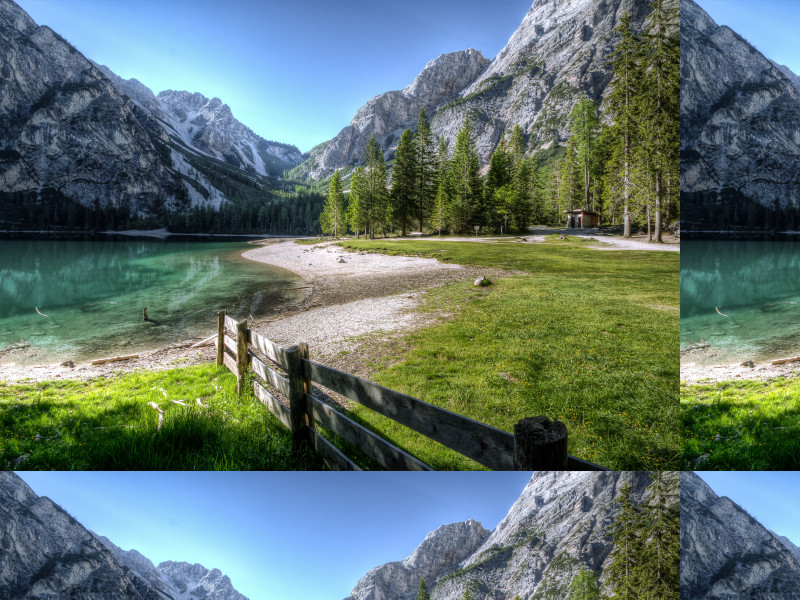\$img->fill(‘tile.png’);

## filter() 指定過濾器

``````// init new image instance
\$img = Image::make('foo.jpg');

// apply filter
\$img->filter(new DemoFilter(45));
``````
``````<?php

namespace Intervention\Image\Filters;

class DemoFilter implements FilterInterface
{
const DEFAULT_SIZE = 10;
private \$size;

public function __construct(\$size = null)
{
\$this->size = is_numeric(\$size) ? intval(\$size) : self::DEFAULT_SIZE;
}

/**
* 執行圖片過濾
*/
public function applyFilter(\Intervention\Image\Image \$image)
{
\$image->pixelate(\$this->size);
\$image->greyscale();

return \$image;
}
}
``````

## width() 圖片寬度

``````// 取得圖片寬度
\$width = Image::make('public/foo.jpg')->width();
``````

## height() 圖片高度

``````// 取得圖片高度
\$height = Image::make('public/foo.jpg')->height();
``````

## interlace() 交錯模式

``````// 建立圖片實例
\$img = Image::make('public/foo.png');

// 啟用 interlacing
\$img->interlace();

// 儲存為 interlaced 的圖片
\$img->save();

// 開啟 interlaced 圖片
\$img = Image::make('public/interlaced.gif');

// 關閉 interlacing
\$img->interlace(false);

// 儲存為 standard mode 的圖片
\$img->save();
``````

## mime() 取得圖片 mime

``````\$mime = Image::make('public/foo.jpg')->mime();
``````

## pickColor() 選出圖片顏色色碼

``````// 建立圖片實例
\$img = Image::make('public/foo.jpg');

// 選出位置在 (100,100) 的像素顏色: 陣列資料
\$arraycolor = \$img->pickColor(100, 100);

/*
array:4 [
0 => 43
1 => 58
2 => 78
3 => 1.0
]
*/

// 選出位置在 (100,100) 的像素顏色: rgb
\$rgb_color = \$img->pickColor(100, 100, 'rgb');
// rgba(43, 58, 78)

// 選出位置在 (100,100) 的像素顏色: rgba
\$rgba_color = \$img->pickColor(100, 100, 'rgba');
// rgba(43, 58, 78, 1.00)

// 選出位置在 (100,100) 的像素顏色: hex
\$hex_color = \$img->pickColor(100, 100, 'hex');
// #2b3a4e
``````

## pixel() 在指定像素點畫出顏色

``````// 建立畫布
\$img = Image::canvas(100, 100, '#ddd');

// 在 (32,32) 像素畫出藍色
\$img->pixel('#0000ff', 32, 32);

// 在 (32,32) 像素畫出紅色
\$img->pixel('#ff0000', 64, 64);
``````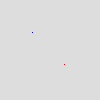\$img->pixel();

## polygon() 畫出多邊形

``````// 建立畫布
\$img = Image::canvas(800, 600, '#ddd');

// 定義多邊形位置
\$points = array(
40,  50,  // Point 1 (x, y)
20,  240, // Point 2 (x, y)
60,  60,  // Point 3 (x, y)
240, 20,  // Point 4 (x, y)
50,  40,  // Point 5 (x, y)
10,  10   // Point 6 (x, y)
);

// 畫出多邊形
\$img->polygon(\$points, function (\$draw) {
// 背景顏色 #000ff
\$draw->background('#0000ff');
// 邊框寬度 1，顏色 #ff0000
\$draw->border(1, '#ff0000');
});
``````

## rectangle() 畫出四邊形

``````// 建立畫布
\$img = Image::canvas(250, 300, '#ddd');

// 畫出紅色四邊形
\$img->rectangle(5, 5, 195, 195, function (\$draw) {
\$draw->background('#ff0000');
});
``````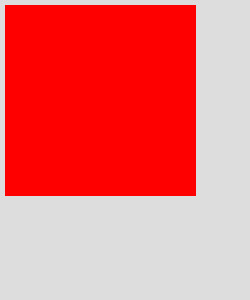\$img->rectangle() background #ff0000

``````// 畫出透明度 0.5 的四邊形，使用 2px 黑色邊框
\$img->rectangle(5, 5, 195, 195, function (\$draw) {
\$draw->background('rgba(255, 255, 255, 0.5)');
\$draw->border(2, '#000');
});
``````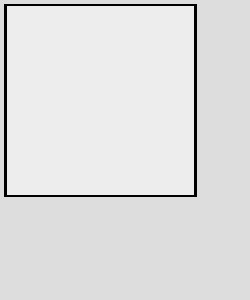\$img->rectangle() background rgba(255, 255, 255, 0.5) border 2px #000

## response() 回應包含 HTTP Header 圖片

• jpg — return JPEG encoded image data
• png — return Portable Network Graphics (PNG) encoded image data
• gif — return Graphics Interchange Format (GIF) encoded image data
• tif — return Tagged Image File Format (TIFF) encoded image data
• bmp — return Bitmap (BMP) encoded image data

• 數值介於 0~100
``````// 建立圖片實例
\$img = Image::make('public/foo.jpg');
return \$img->response();

// 回應 png 圖片
return \$img->response('jpg');
``````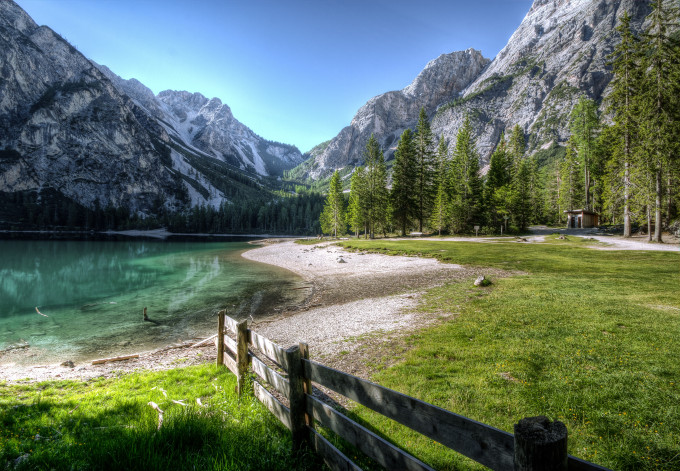\$img->response(‘jpg’);

``````// 回應 jpg 圖片 品質 20
return \$img->response('jpg', 20);
``````\$img->response(‘jpg’, 20);

Laravel 是 PHP 的框架（Framework），提供了很多開發網站或 API 所需的工具及環境，經過簡單的設定就可以完成資料的處理及顯示，使開發者可以很優雅且快速的開發出各個不同的產品。本書適合有 PHP 基礎的人，但不知道要怎麼選擇框架，或者不用框架的人也能夠明白它的好處。### 購書連結